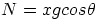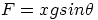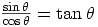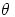## A Simple Experiment, goin not so simply! Determination of the coefficient of friction

### tonyvers

Posted 08 February 2007 - 08:57 PM

Hello there,

As you have probably guessed, i am stuck on this experiment. To cut a long story short,
my aim is to determine the coefficient of friction. So any help would be appreciated. Basically, i have a block of wood (X grams) and an inclined plane which i can adjust height. So my question is what do i do next? I am lifting the inclined plane until the block of wood begins to slide down. At the same time i record the angle that this occurs. But i don't know what to do next to find the coefficient. I knw tht F/N = μ but i can't see how that relates to my case. Please help, id be very grateful.

Thanks
Tony

Posted 08 February 2007 - 09:08 PM

Okay, well on the point of slipping:Whereis the coefficient, N is the normal reaction of the plane on the object and F is the frictional force acting up the plane.

Now, since there is no motion, we can say that the forces are balanced. Resolving the forces perpendicular to the plane we get:(the component of the weight acting into the plane equals the reaction force)

and resolving parallel to the plane we get:(the frictional force up the plane is equal to the component of the weight acting down the plane)

Can you see how to use these in the equation you posted?

### tonyvers

Posted 08 February 2007 - 09:15 PM

Thanks for the speedy reply! yeah that helps a lot!! So just to clarify i could do μ=F/N which is the same as μ = xgsinθ/xgcosθ ?? and then jus replace with numbers. I know this is a bit simple but would i change x to the weight of the block? g to 9.8 and θ to the angle it begins to slide? Thanks again..
TOny

Posted 08 February 2007 - 09:33 PM

Thanks for the speedy reply! yeah that helps a lot!!
You're welcomeQUOTE
So just to clarify i could do μ=F/N which is the same as μ = xgsinθ/xgcosθ ?? and then jus replace with numbers. I know this is a bit simple but would i change x to the weight of the block? g to 9.8 and θ to the angle it begins to slide? Thanks again..
TOny
Yeah, you've got the right idea for x,g, and. However, this isn't actually too important, the equation can be simplified:the "xg" can cancel from this fraction as it is in the numerator and the denominator, and also, the trigonometric identitycan be used, giving:Which is quite interesting, because the angle at which it starts sliding is completely independent of the mass!

### tonyvers

Posted 08 February 2007 - 09:37 PM

haha cool! so it really is pretty simple. To find μ i just find the angle and take its tan?! thanks!

### dfx

Posted 08 February 2007 - 10:09 PMIn the above formula,is known as the "angle of friction" and is the angle that the resultant of the Friction force and the Normal reaction makes with the inclined plane. But that's stretching it a little, just in case you're interested. As you can see,will vary depending on the magnitude of the friction force and traces out what's known as a "cone of friction" upto the point of slipping.

### Blondie

Posted 12 February 2007 - 06:49 AM

That sounds horribly familiar. I hate investigations. >.<